# RBI Assistant Mains 2022 Quantitative Aptitude Quiz – 6

## RBI Assistant 2022 Quantitative Aptitude Quiz

Quantitative Aptitude measures a candidate’s numerical ability and problem-solving abilities. It is the most important section of almost all competitive exams. Candidates are often stymied by the complexity of Quantitative Aptitude Questions but if they practice more and more questions, it will become quite simple. So, here we are providing you with the RBI Assistant Mains 2022 Quantitative Aptitude Quiz to enhance your preparation for your upcoming examination. This RBI Assistant Mains 2022 Quantitative Aptitude Quiz is based on the most recent and the latest exam pattern. A detailed explanation for each question will be given in this RBI Assistant Mains 2022 Quantitative Aptitude Quiz. This RBI Assistant Mains 2022 Quantitative Aptitude Quiz is entirely free of charge. This RBI Assistant Mains 2022 Quantitative Aptitude Quiz will assist aspirants in achieving a good score in their upcoming examinations.

Directions (1-5): What will come in the place of the question mark (?) in the following number series?
1. 9.8, ? , 10.6, 7.4, 13.8, 1
(a) 10
(b) 9
(c) 12
(d) 18
(e) 21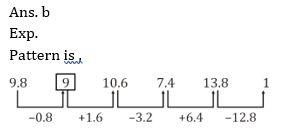2. 1, 3, 21, 71, ? , 331
(a) 209
(b) 201
(c) 189
(d) 161
(e) 1693. 8, 5, 6, 10, ? , 53.5
(a) 18
(b) 23.5
(c) 21
(d) 32
(e) 36.5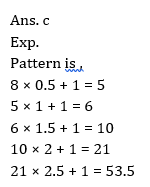4. 1, 3, 12, 40, ? , 231
(a) 95
(b) 98
(c) 105
(d) 112
(e) 1185. 7, 20, 46, 98, 202, ?
(a) 410
(b) 408
(c) 398
(d) 310
(e) 520Directions (6-10): The following questions are accompanied by two statements A and B. You have to determine which statements(s) is/are sufficient/necessary to answer the questions.
6. A box contains 3 Green balls, 5 blue balls and X red balls. Find the value of X ?
A) Probability of choosing one ball from the box which is red is 1/3.
B) Probability of choosing one ball from the box which is green is 1/4
(a) Statement A alone is sufficient to answer the question but statement B alone is not sufficient to answer the questions.
(b) Statement B alone is sufficient to answer the question but statement A alone is not sufficient to answer the question.
(c) Both the statements taken together are necessary to answer the questions, but neither of the statements alone is sufficient to answer the question.
(d) Either statement A or statement B by itself is sufficient to answer the question.
(e) Statements A and B taken together are not sufficient to answer the question.7. Cylinder ‘A’ is melted and casted into another cylinder ‘B’. Find out the height of cylinder ‘A’ ?
A) Height of cylinder ‘A’ and cylinder ‘B’ is same. while base radius of cylinder ‘B’ is 6 cm.
B) Curved surface area of cylinder ‘A’ is 528/7 more than circumference of base circle of cylinder ‘A’
(a) Statement A alone is sufficient to answer the question but statement B alone is not sufficient to answer the questions.
(b) Statement B alone is sufficient to answer the question but statement A alone is not sufficient to answer the question.
(c) Both the statements taken together are necessary to answer the questions, but neither of the statements alone is sufficient to answer the question.
(d) Either statement A or statement B by itself is sufficient to answer the question.
(e) Statements A and B taken together are not sufficient to answer the question.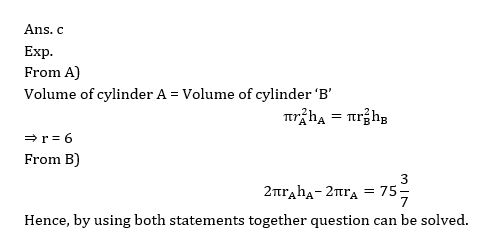8. ‘A’ started a business. B joined him after 3 months and C joined them after 4 months. Find the share of ‘C’ out of total profit?
A) ‘A’ invested 6000 more than ‘B’ while ‘B’ invested 3000 more than ‘C’
B) A’s profit is Rs. 32,000 out of total profit.
(a) Statement A alone is sufficient to answer the question but statement B alone is not sufficient to answer the questions.
(b) Statement B alone is sufficient to answer the question but statement A alone is not sufficient to answer the question.
(c) Both the statements taken together are necessary to answer the questions, but neither of the statements alone is sufficient to answer the question.
(d) Either statement A or statement B by itself is sufficient to answer the question.
(e) Statements A and B taken together are not sufficient to answer the question.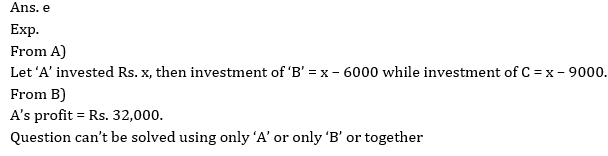9. If ‘x’ is a positive integer. Is ‘y’ a positive integer?
A) HCF and LCM of ‘x’ and ‘y’ is 5 and 195 respectively.
B) Average of ‘x’, ‘y’ and ‘y+1’ is an integer.
(a) Statement A alone is sufficient to answer the question but statement B alone is not sufficient to answer the questions.
(b) Statement B alone is sufficient to answer the question but statement A alone is not sufficient to answer the question.
(c) Both the statements taken together are necessary to answer the questions, but neither of the statements alone is sufficient to answer the question.
(d) Either statement A or statement B by itself is sufficient to answer the question.
(e) Statements A and B taken together are not sufficient to answer the question.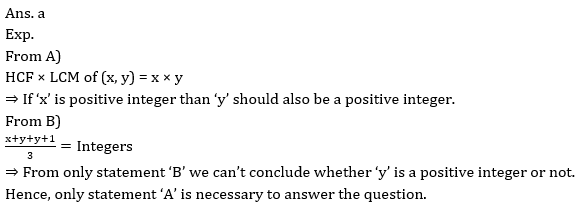10. Ronit sell a pen to Abhishek at 50% profit. Find the cost price of pen at which Ronit bought it?
A) Abhishek marked the price 60% above the cost price and at selling time gave discount of 20% and earns 28% profit.
B) Abhishek sell the pen to Neeraj at Rs. 390 and earn 30% profit.
(a) Statement A alone is sufficient to answer the question but statement B alone is not sufficient to answer the questions.
(b) Statement B alone is sufficient to answer the question but statement A alone is not sufficient to answer the question.
(c) Both the statements taken together are necessary to answer the questions, but neither of the statements alone is sufficient to answer the question.
(d) Either statement A or statement B by itself is sufficient to answer the question.
(e) Statements A and B taken together are not sufficient to answer the question.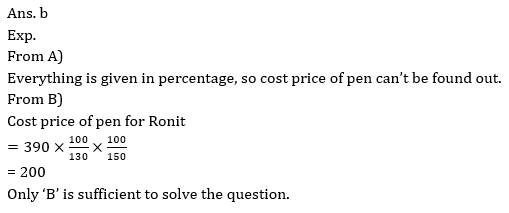###Click to Buy Bank MahaCombo Package

Recommended PDF’s for:

#### Most important PDF’s for Bank, SSC, Railway and Other Government Exam : Download PDF Now

AATMA-NIRBHAR Series- Static GK/Awareness Practice Ebook PDF Get PDF here
The Banking Awareness 500 MCQs E-book| Bilingual (Hindi + English) Get PDF here
AATMA-NIRBHAR Series- Banking Awareness Practice Ebook PDF Get PDF here
Computer Awareness Capsule 2.O Get PDF here
AATMA-NIRBHAR Series Quantitative Aptitude Topic-Wise PDF Get PDF here
AATMA-NIRBHAR Series Reasoning Topic-Wise PDF Get PDF Here
Memory Based Puzzle E-book | 2016-19 Exams Covered Get PDF here
Caselet Data Interpretation 200 Questions Get PDF here
Puzzle & Seating Arrangement E-Book for BANK PO MAINS (Vol-1) Get PDF here
ARITHMETIC DATA INTERPRETATION 2.O E-book Get PDF here
3